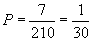Understanding Probability: How to Calculate the Number of Outcomes

Key Terms

o       Counting problem

o       Replacement

o       Permutation

o       Combination

Objectives

o       Understand how to relate counting of outcomes to probability

o       Calculate the number of outcomes of a random experiment using permutations and combinations

o       Know how sampling with or without replacement affects a counting problem

When solving more complicated probability problems, we may need to consider series of random experiments or experiments that involve several different aspects, such as drawing two cards from a deck or rolling several dice. In such cases, the ability to calculate relative frequencies (and thus probabilities) requires counting the number of possible outcomes of the experiment. Although counting the number of possible outcomes for simple random experiments, such as the flip of a coin (heads or tails), may be quite simple, counting the number of possible outcomes for the ordered selection of three cards from a standard deck may not be. We will show you how to handle these more complicated counting problems, thereby expanding your ability to solve a range of statistics problems.

Replacement and Ordering

One crucial aspect of many counting problems in probability is whether replacement is used when sampling. For instance, we may be inquiring about whether a drawing of a name from a hat is statistically "fair"; to do so might require performing a series of trials where a name is pulled from the hat. The question arises as to whether that name should be returned to the hat after being drawn: if it is returned, this selection is called sampling with replacement; if not, then it is called sampling without replacement. In the example case of drawing a name from a hat, the drawn name would be returned to the hat for the following trial so that the conditions of each drawing are the same--thus, this example would involve sampling with replacement.

Another aspect of counting problems is the applicability of order for the results of the experiment or experiments. In some cases, we may be interested in an ordered set of results: for example, the number of possible combinations for a padlock. On the other hand, we might be interested in a set of results for which order is not relevant, such as how many five-card hands can be dealt from a standard deck. Order is thus another aspect of counting problems that you should keep in mind when solving them.

Permutations

Let's say we have a set of n objects (such as playing cards, for instance), and we want to make k selections, with replacement, while taking note of the order. We can derive a formula for this case by imagining how we would go about such an experiment. Because we return the object after each selection, every trial has n objects and therefore n potential outcomes. In the first trial, there are n possible outcomes. In the second trial, there are likewise n possible outcomes, resulting in n(n) = n2 different outcomes for the two successive trials. Following this pattern, if we make k selections with replacement from n objects, there are nk possible outcomes, where the order of the outcome is important.

Practice Problem: An opaque bag holds five tiles marked with the letters A, B, C, D, E. If three random selections are made from this bag (with the tile being returned to the bag after each selection), how many different words can be formed? (Assume that a "word" need not be a standard English word.)

Solution: This problem involves sampling with replacement, and the order of the results is important (although DEA and ADE both use the same letters, for example, they are not the same word). One approach is to attempt to list each possible word:

AAA

AAB

AAC

Interested in learning more? Why not take an online Statistics course?

and so on. This approach, although conceptually correct, is lengthy and tedious. Let's use the approach discussed above. In each drawing, we have five potential results: A, B, C, D, or E. Since we are drawing a tile three successive times, the number of possible outcomes is 53, or 125. Obviously, writing out all the possible words would be time-consuming indeed! Our counting method saves us a significant amount of time, however.

For the case of k selections from n objects, without replacement but still taking note of order, we want to calculate the number of permutations. We can use the following formula, where the number of permutations of n objects taken k at a time is written as nPk. The factorial notation (!) is also defined below.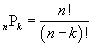where x! = x

(x – 1)

(x – 2) .

2

1

To understand how we get this formula, first consider the case where we want to find how many ways we can order all n objects. The first choice allows us n options, the second choice allows us n – 1 options, the third choice allows us n – 2 options, and so on, all the way down to 1. The total number of possible orderings is the product of all these numbers, which we can write as n!. If we only make k selections, then we must choose from n objects, then n – 1 objects, and so on, down to n – k + 1. But this just leads us to the formula for permutations given above, which is illustrated in the following practice problem.

Practice Problem: A group of five horses is racing at a track. How many different ways can the horses place in the top three?

Solution: We can calculate the number of ways the horses can place in the top three by calculating the number of permutations. Let's also consider the problem from a more fundamental perspective. For first place, there are five different possible horses. For each of these possibilities, there are four remaining possibilities for second place-we then multiply five and four. For third place, we have three remaining possibilities for each of the preceding results--calculate the product of five, four, and three, which is 60. We don't care about the last two places. Note how the formula for permutations is related to our fundamental approach to the problem: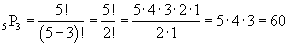Thus, the result is 60.

Combinations

Some problems require us to calculate a number of possible outcomes without respect to ordering. Such a case is a lottery drawing where all that is required to win is to pick the correct numbers in any order. This problem requires us to make a change to the formulas above so as to disregard cases that have the same set of objects, but with a different ordering.

Consider the case where selection is made without replacement. The number of permutations, nPk, uses the formula given above. We can alter this formula to disregard ordering by eliminating each ordering of each set of objects. Since we are choosing k objects from a set of n objects, those k objects can be ordered in k! ways (see our previous discussion). So, if we simply divide nPk by k!, we then have the number of ways we can select k objects from n without replacement and without regard for order. This is called the number of combinations of n taken k at a time, which is sometimes written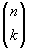.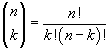Practice Problem: There are five remaining cards from a standard deck. A player must draw two of them. How many different hands can he draw?

Solution: This problem requires us to calculate the number of combinations of five cards taken two at a time. Note that order is unimportant and that drawing the cards cannot involve replacement. We can take the exhaustive and tedious approach of writing out all the possibilities as follows, where we label the cards A, B, C, D, and E.

AB BC CD DE

AC BD CE

AE

This approach indicates that there are 10 possible combinations of 5 cards taken 2 at a time. If we use the combinations formula, we get the same result.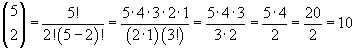If the problem required us to calculate a much larger number (such as if the player had to select 2 cards from a full deck of 52), then writing out all the possibilities would be unduly time consuming. The formula, however, works in every case.

Although we will not consider the case of combinations with replacement in depth, the formula can be shown to be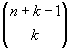. This result can be proven, or you can simply try a few simple cases to demonstrate that it works.

Relating Probability and Counting

Now that we can count the number of possible outcomes of various types of random experiments, we can also calculate the relative frequencies (and therefore probabilities) of certain events. To do so (assuming each outcome is equally probable, which is not always the case), simply divide the number of outcomes in the event of interest by the total number of possible outcomes. Thus, if we want to calculate the probability of drawing an ace from a standard deck of playing cards, we can divide the number of outcomes in the event where an ace is drawn (4) by the total number of possible outcomes where any card is drawn (52). The probability is then 1/13. Thus, the counting skills discussed above allow us to calculate the probabilities associated with a variety of problems.

Practice Problem: A certain lottery has a hat with the numbers 1 through 10 each written on a single scrap of paper. Three numbers are successively pulled from the hat and set aside in no particular order. If a player can pick four numbers, what is the probability that he wins the lottery?

Solution: First, consider the selection of the winning numbers in the lottery. We know that the numbers are not replaced after they are chosen and that the order of the selection is not important; thus, the winning lottery numbers are three unique numbers. Now, we want to calculate a player's chance of winning the lottery if he is able to pick four numbers. The winning combination includes the three numbers chosen in the lottery as well as an additional fourth number, which can be any of the remaining numbers. Apart from the three winning numbers, there are seven other numbers that can be chosen for the fourth number. As a result, the player has seven possible winning combinations. To calculate the probability of winning, we must now find out how many total combinations of 4 numbers can be chosen from 10; to do so, we can use the combinations formula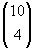.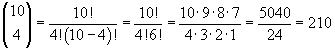The probability P of the player winning is thus